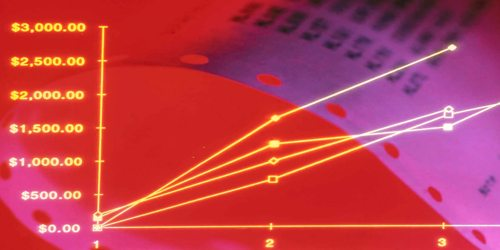Accounting

# General Depreciation System (GDS)The general depreciation system (GDS) for measuring depreciation is the most widely used modified accelerated cost recovery system (MACRS). This works within a period of recovery which is shorter than in the Alternative Depreciation System (ADS). GDS effectively uses the diminishing balance approach to depreciate personal property. It is the foremost common method when it involves calculating the depreciation of assets under the tactic of MACRS. For tax purposes, the MACRS is that the primary method of depreciation and uses either the declining balance method or the straight-line method.

Specific asset methods and lifetimes exist that help to measure the tangible tax deductions for property depreciation. Usually, assets are classified into groups by form or sector in which that particular asset is used. The method of decreasing balance involves applying the rate of depreciation against the non-depreciated balance. For example, if an asset that costs \$1,000 is depreciated at 25% annually, the deduction is \$250 within the first year and \$187.50 within the second year, and so on.General Depreciation System (GDS)

In general, GDS is the most common method for measuring asset depreciation under the MACRS method. Although the residential and non-residential real property is depreciated using the straight-line process, personal property uses the form of declining-balance to be refused. The MACRS system of depreciation allows for larger depreciation deductions within the early years and lower deductions within the later years of ownership.

Since GDP uses the declining-balance approach let’s look at how it functions in the example: Let’s assume that an asset costing \$1,000 undergoes a 25 percent depreciation per year. The deduction in year one is \$250.00 and then in year two is \$187.50 and so forth. Under MACRS, a taxpayer must use defined asset lives and methods to measure tax deductions for depreciation of tangible real property. There are two MACRS sub-systems: the general depreciation system (GDS), and the alternative system of depreciation (ADS). GDS is the most important and is the most commonly used asset.

Each method of depreciation differs with regard to the number of years the asset might be depreciated across. Generally, the GDS uses shorter recovery times compared to the ADS. And, each year, the latter one sets the depreciation as an equivalent number, except for the first and last year, which may not be a full 12-year cycle. However, certain assets have an identical recovery period under either system. As an example, cars, some trucks, and computers are depreciated over five years irrespective of the system employed.

There are also unique assets that have a common recovery time when implemented to one of the two schemes. Relevant assets in all of these systems come with the same recovery time. For instance, computers, trucks, cars, and more get depreciated over a period of 5 years, no matter the employed system that they need. The recovery period of those assets is 5 years if applied under either of the systems.

The accelerated methodologies of depreciation and the selection of GDS or Advertising systems may have a material effect on the financial results published. But make sure the Advertising system is used in a certain class with all the properties. If this system is not chosen for a certain asset, then the GDS system cannot be used later. IRS asset classes allocate class life on the basis of various estimates of the life of the asset under the ADS and GDS systems.

Information Sources: